# Update of WordPress

Deutsch

The update of WordPress and its Plugins did not really work.

A small change helped a lot:

In .htaccess put the following line to the beginning of the file:
 AddHandler php56-cgi .php 

Then all updates seemed to work fine.

Maybe this helps others observing the same problem.

# Aktualisierung von WordPress

English

Die Aktualisierung von WordPress und Plugins hat hier nicht so gut funktioniert.

Es hat eine kleine Änderung geholfen, jetzt ist alles gut:

In .htaccess am Anfang diese Zeile
 AddHandler php56-cgi .php 
einfügen…

Vielleicht hilft das auch anderen, die damit Probleme hatten….

# Find the next entry in a sequence

In Facebook, Xing, Google+, Vk.com, Linkedin and other of these social media networks we are often encountered with a trivial question like this:
 1->2 2->8 3->18 4->32 5->50 6->72 7->? 
There are some easy patterns. Either it is some polynomial formula or some trick with the digits.
But the point is, that any such sequence can easily be fullfilled by a polynomial formula. That means we can put any value for 7 and make it work. Or any answer is correct. So what would probably be the real question is the most simple function to full-fill the given constraints. Simplicity can be measured in some way… If the solution is unique is unclear, but let us just look at the polynomial solution.

A function is needed that takes as parameter a list of key-value-pairs (or a hash map) and that yields a function such that the function of any of the key is the associated value.

Assuming a polynomial function in one variable we can make use of the chinese remainder theorem, which can be applied to univariate polynomials over a fieldas well as to integral numbers. For a polynomial p(X) we have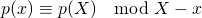where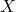is the polynomial variable and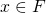is a concrete value.

We are looking for a polynomialsuch that for given valueswe have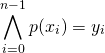or in another way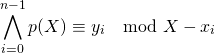which is exactly the Chinese remainder theorem.
LetandWe can see that for all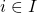the polynomials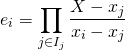have the properties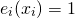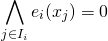or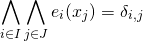where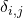is the Kronecker symbol, which is 0 if the two indices differ and 1 if they are equal.
Or as congruence: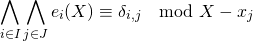Then we can just combine this and use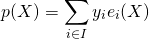This can easily be written as a Ruby function
 def fun_calc(pairs)   n = pairs.size   result = lambda do |x|     y = 0     n.times do |i|       p_i = pairs[i]       x_i = p_i.to_r       y_i = p_i.to_r       z = y_i       n.times do |j|         if (j != i)           p_j = pairs[j]           x_j = p_j           z *= (x - x_j) / (x_i - x_j)         end       end       y += z     end     y   end   result end 
This takes a list of pairs as a parameter and returns the polynomial function als lambda.
It can be used like this:
 lop = [[0, 0], [1, 1], [2, 4], [3, 9], [4, 16], [5, 25], [6, 36], [7, 64]]

 f = fun_calc(lop) 

20.times do |x|   y = f.call(x)   puts sprintf("%6d -> %6d", x, y) end 
Put this together into a ruby program and add some parsing for the list of pairs or change the program each time you use it and all these „difficult“ questions „that 99.9% fail to solve“ are not just easy, but actually soluble automatically.

This is interesting for more useful applications. I assume that there will always be situations where a function is needed that meets certain exact values a certain inputs and is an interpolation or extrapolation of this.

Please observe that there are other interesting and useful ways to approach this:

• Use a „best“ approximation from a set of functions, for example polynomials with a given maximum degree
• use cubic splines, which are cubic polynomials within each section between two neighboring input values such that at the input values the two adjacent functions have the same value (, of course), the same first derivative and the same second derivative.

For highway and railroad construction other curves are used, because the splines are making an assumption on what is the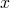-axis and what is the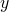-axis, which does not make sense for transport facilities. They are using a curve called Clothoid.

Use Java, C, Perl, Scala, F# or the programming language of your choice to do this. You only need Closures, which are available in Java 8, F#, Scala, Perl, Ruby and any decent Lisp dialect. In Java 7 they can be done with an additional interface as anonymous inner classes. And for C it has been described in this blog how to do closures.

The idea of threads is to share almost all information between the threads of the same process. This implies more effort to avoid creating inconsistencies by simultaneous access to the same data. But sometimes it is necessary to have data private to one thread. This is usually easily accomplished by just using local variables and method or function parameters that are simply not naturally available to the other threads, unless they are referenced by some global structures or by structures shared with other threads. But there are some cases when it is useful to have thread local variables.

The general idea is to call a function or method several times and to retain intermediate results somewhere. In object oriented programming attributes may be used for this, in procedural programming global variables or a structure that is passed around. In functional programming this is just forbidden and a workaround is always possible but this may be painful, complicated, inefficient or impossible due to the usage of existing functionality written by others.

Now the global variable fails miserably, one of the reasons, it should not be used. And its object-oriented blessed brother, like a static attribute in Java or C++, as well. Multiple threads may run simultanous sessions with these functionalities. So thread local variables are the better approach.

They can easily be built manually. You just need to use a hash map, make sure its access is thread safe by using RWLocks in C or ConcurrentHashMap or some synchronized HashMap in Java. Then use the thread ID as key to the hash map and store some structure in it where all the bad stuff is stored. Or use multiple hash maps.

But both languages provide better facilities for this purpose. In newer Java versions ThreadLocal should be used. This needs an initializer. Then it provides a get method that accesses the thread local copy of the variable and creates it, if it is not present. This approach is strongly recommended when dealing the DateFormat and NumberFormat, which are not thread safe in Java. Using synchronized on the format or creating it on demand each time works as well, but the thread local approach seems to be the most strait-forward and most efficient way. But these special examples should lose their importance. Using Joda-Time or the new Java-8 functionality for Date and Time should make SimpleDateFormat, DateFormat, Calendar and java.util.Date obsolete. And NumberFormat is still useful, but in many cases replaceable by String.format() and the C-like functionality.